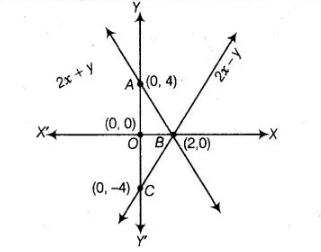# Write a pair of linear equations

Question:

Write a pair of linear equations which has the unique solution x = – 1 and y = 3. How

many such pairs can you write?

Solution:

Condition for the pair of system to have unique solution

$\frac{a_{1}}{a_{2}} \neq \frac{b_{1}}{b_{2}}$

Let the equations are,

$a_{1} x+b_{1} y+c_{1}=0$

and

$a_{2} x+b_{2} y+c_{2}=0$

Since, $x=-1$ and $y=3$ is the unique solution of these two equations, then

$a_{1}(-1)+b_{1}(3)+c_{1}=0$

$\Rightarrow \quad-a_{1}+3 b_{1}+c_{1}=0$ $\ldots$ (i)

and $\quad a_{2}(-1)+b_{2}(3)+c_{2}=0$

$\Rightarrow \quad-a_{2}+3 b_{2}+c_{2}=0 \quad \ldots$ (ii)

So, the different values of $a_{1}, a_{2}, b_{1}, b_{2}, c_{1}$ and $c_{2}$ satisfy the Eqs. (i) and (ii).Hence, infinitely many pairs of linear equations are possible.